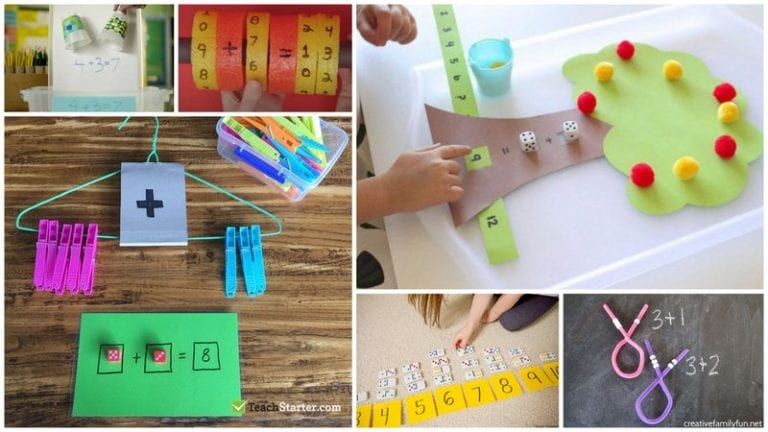# 28 Subtraction Activities That Are Nothing Less Than Awesome

Your students will love these hands-on ideas.

Learning subtraction takes practice, just like any math skill. So it’s a good idea to have a whole collection of clever subtraction activities in your toolbox. These are some of our favorites for the classroom or to send home with students to practice at home.

(Just a heads up, WeAreTeachers may collect a share of sales from the links on this page. We only recommend items our team loves!)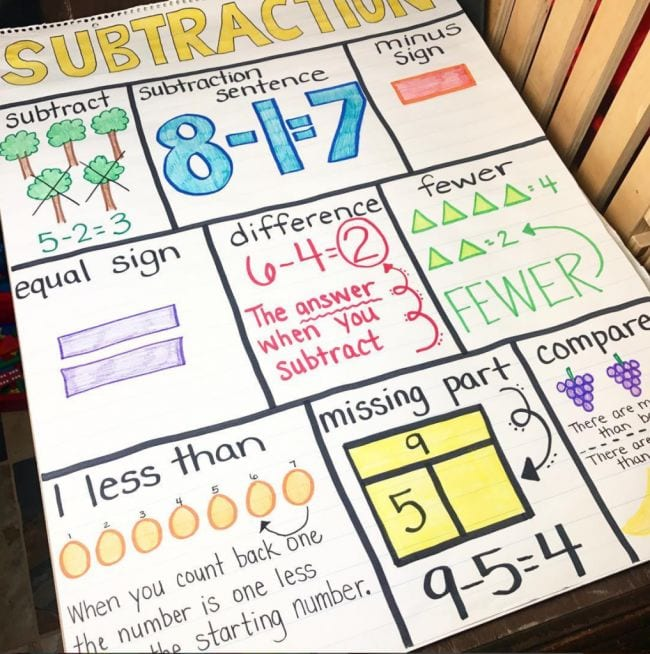Anchor charts give kids something to refer back to as they work on new concepts and skills. We like this subtraction anchor chart for all the details it provides.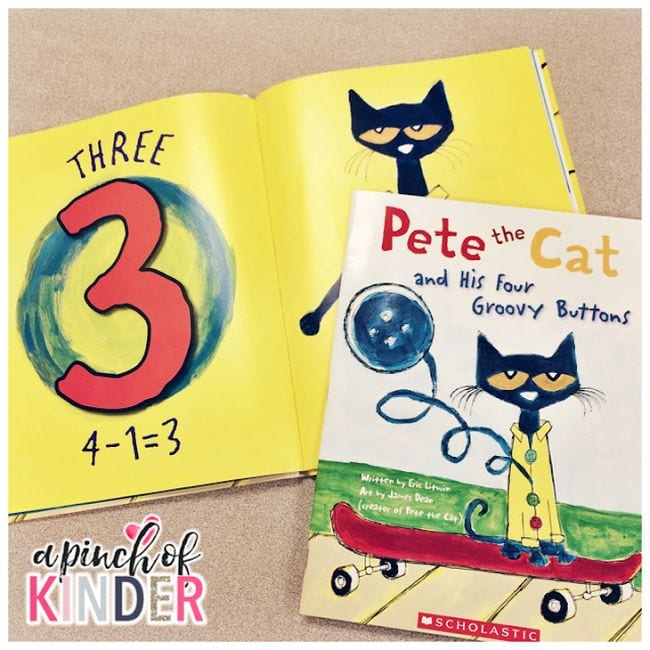Read-alouds are such a fun way to introduce new math concepts to kids. There are lots of great books to work into your subtraction activities, like our perennial favorite Pete the Cat and His Four Groovy Buttons.

## 3. Count Pete’s buttons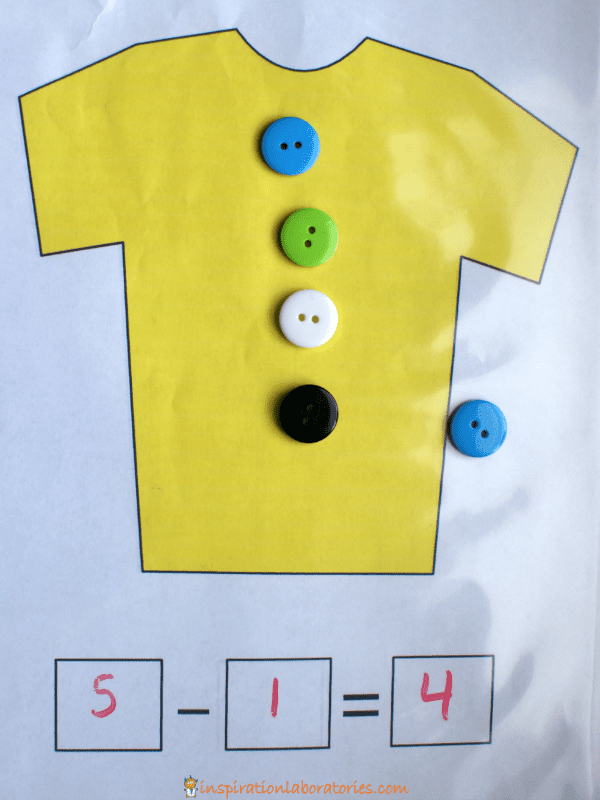If your students love the book, try this idea that incorporates real buttons into subtraction practice. So fun!

## 4. Climb the monkey tree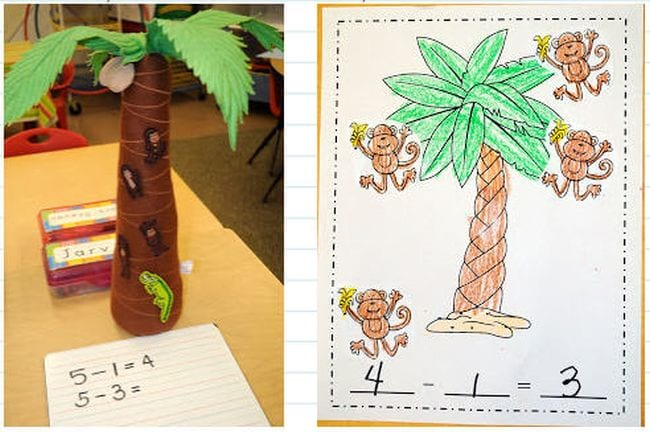Five Little Monkeys is another beloved book with math concepts worked right into the story. Build your own tree for monkeys to climb, or use a doll bed for jumping!

## 5. Try story problems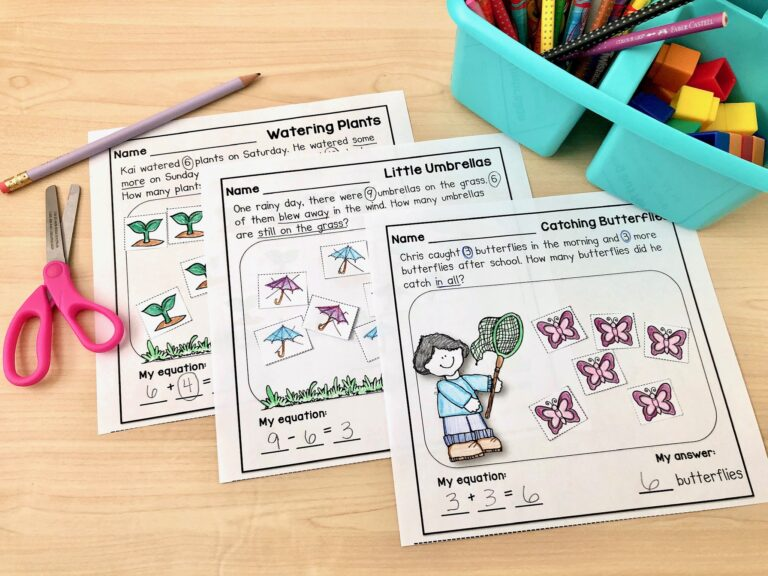One of the best ways to make math meaningful for students is by teaching them how to solve story problems. When they see math in real-world scenarios, it helps numbers make sense in their minds.

## 6. Introduce different strategies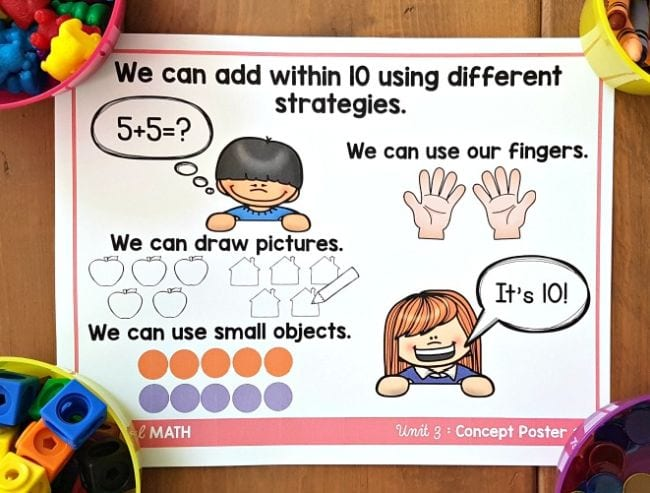Give kids plenty of strategies for understanding subtraction. They can use these when working on subtraction activities and exercises.

## 7. Count with bears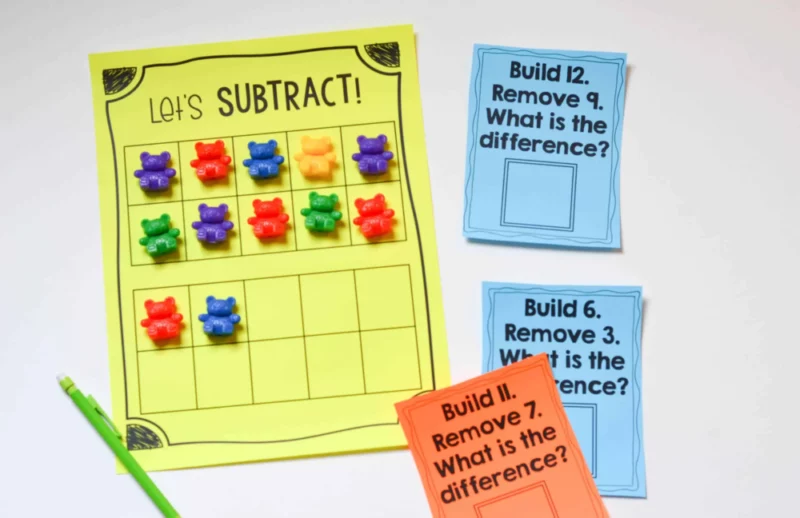Using manipulatives is a great first strategy to use. It is concrete and students can really see what it means to subtract and take away from something.

## 8. Smash some Play-Doh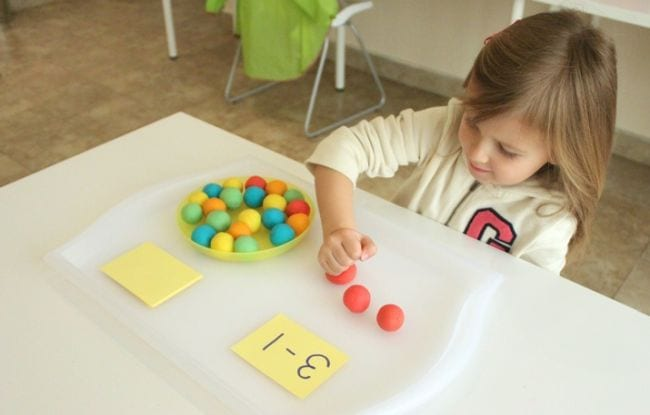For some reason, kids simply love to smash stuff! Roll balls of Play-Doh and let kids smoosh them as they subtract. (Find more ways to use Play-Doh for learning here.)

## 9. Whack-a-ball to subtract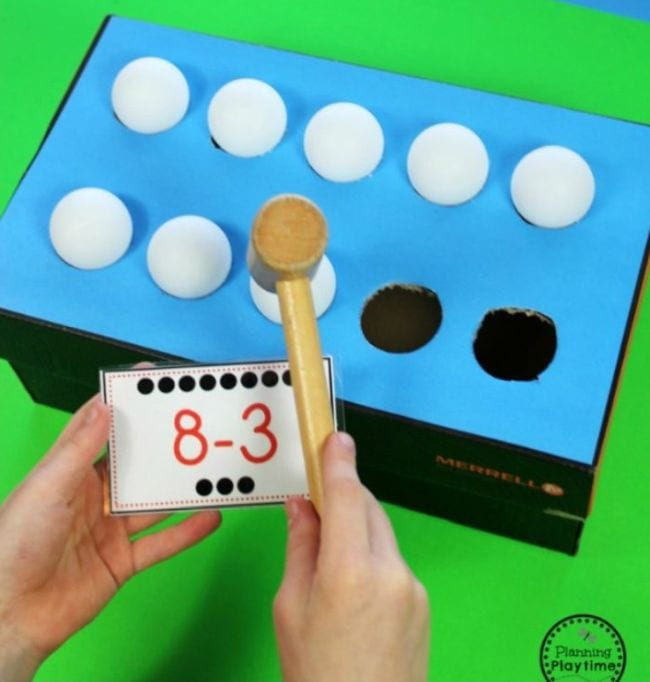Here’s another subtraction activity that lets kids burn off some energy. Make a 3D ten-frame by cutting holes in a shoebox. Rest Ping-Pong balls on the holes, then whack them with a toy hammer to subtract.

## 10. Feed a hungry penguin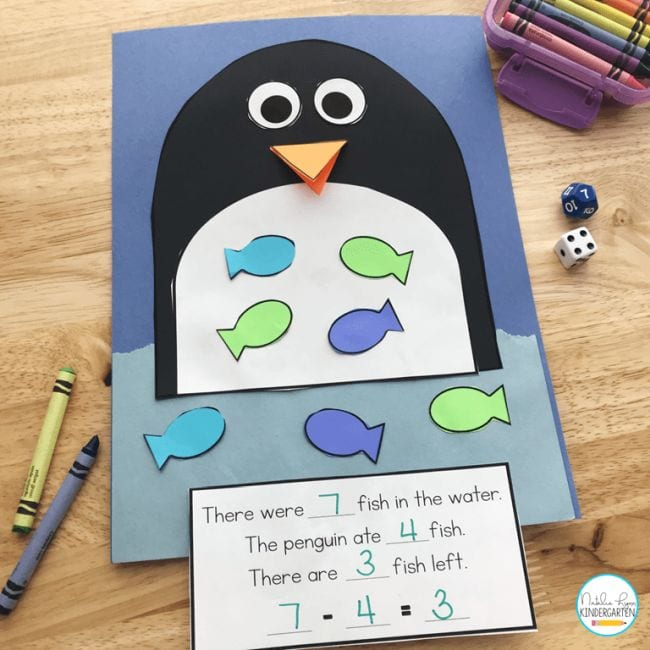This hungry penguin is here to help your students learn how to subtract! Feed him paper fishies and write out the equations.

## 11. Visualize the problem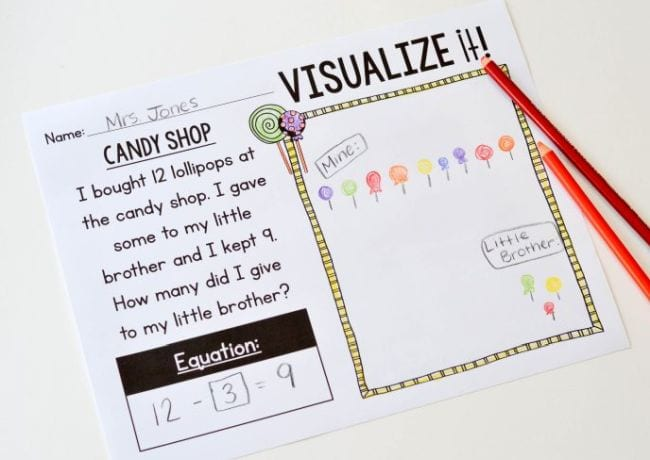Subtraction activities like story problems are easier when kids can visualize the action. Try drawing pictures or acting out the story to make the concept real.

## 12. Sort flash cards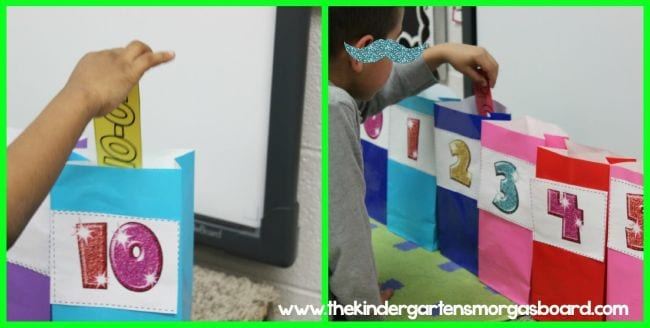Label a row of paper bags 1 to 10, then sort flash cards into the correct bags. Make it a timed exercise and have two teams race to see who’s the fastest (and most accurate)!

## 13. Bowl and subtract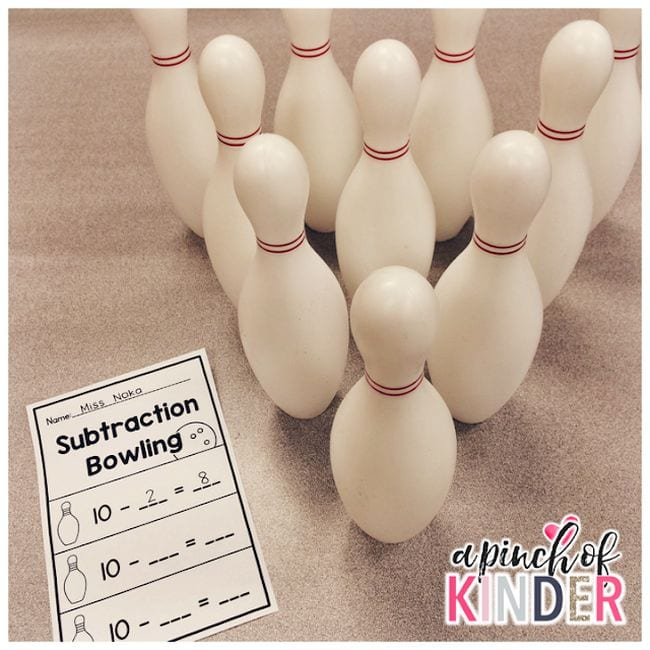This is one of the most popular subtraction activities out there. Kids bowl, knock down pins, and subtract them from the total. This is too much fun to be learning, right?

## 14. Roll and Race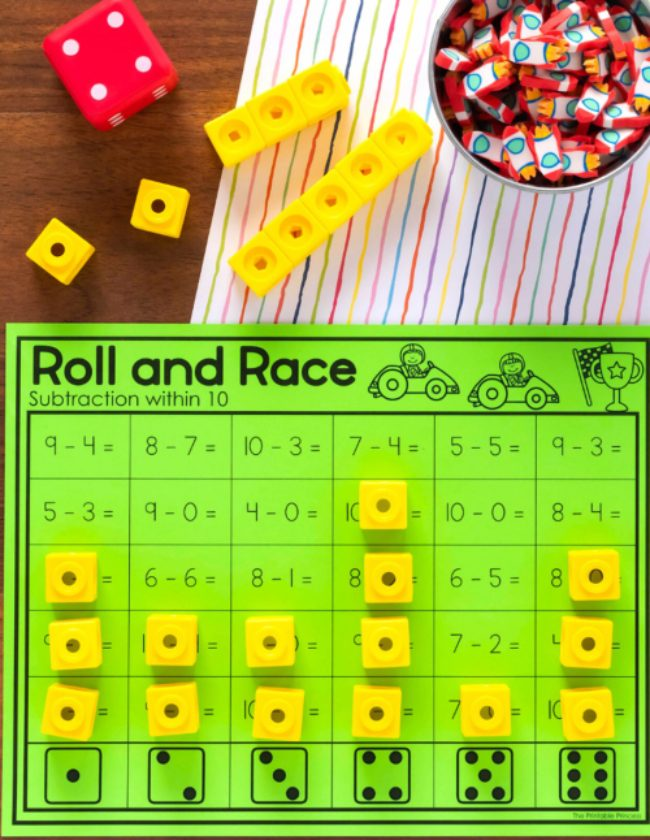Students roll a die and match the number to the column on their game board. Then they solve the first available problem, either coloring in or covering the space with a manipulative. The first player to the top of a column wins.

## 15. Have a LEGO subtraction race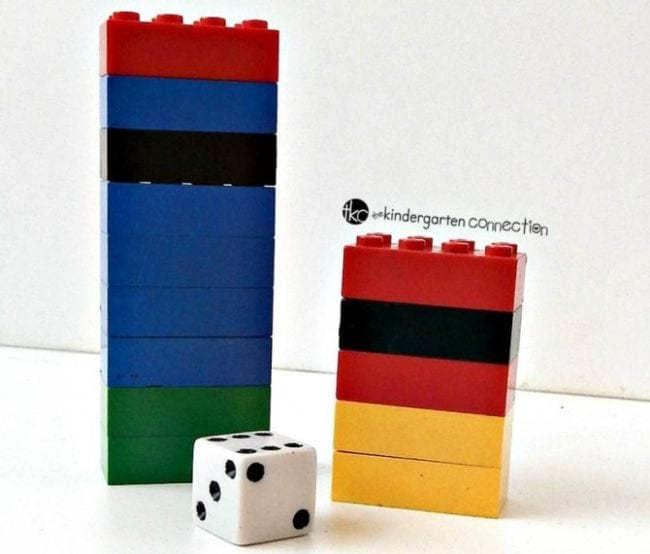Each person starts with a stack of LEGO bricks. Roll the die and take away the number shown, stating the equation out loud each time. First to zero wins!

## 16. Find the key to the lock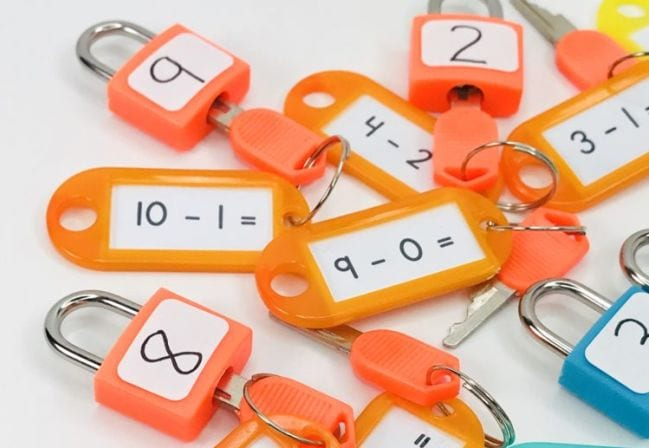Invest in a set of locks that you can use for all kinds of things, including subtraction activities. These 8-packs from Amazon come in a variety of colors and should last for years.

## 17. Use LEGO bricks for double digits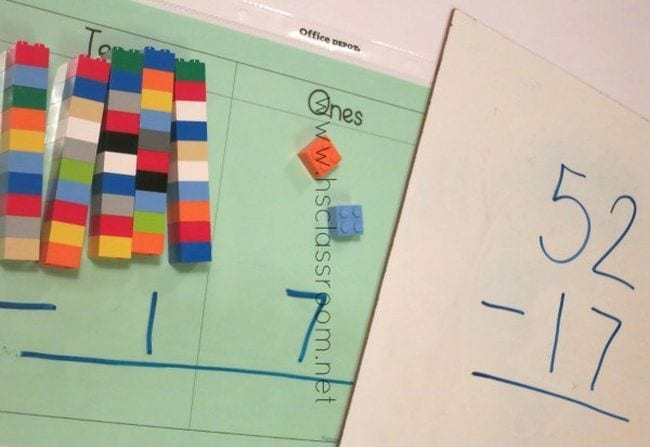Ready to talk about “borrowing” and double-digit math? Make the concept easier by using LEGO bricks.

## 18. Grab a Hula-Hoop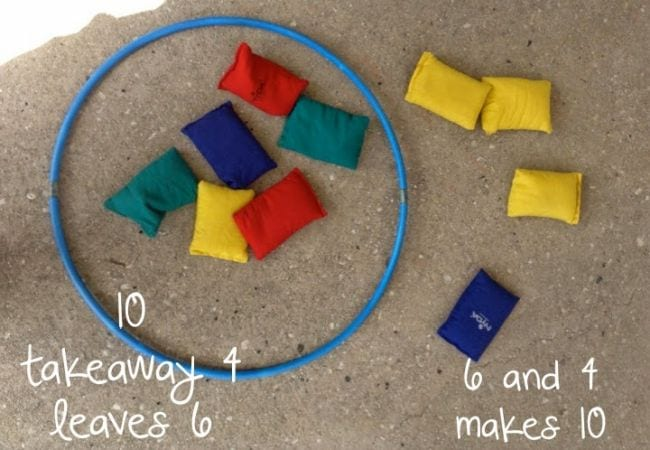Start by tossing bean bags and seeing how many you can get into the hoop. Then, turn the results into subtraction equations.

## 19. Ask “How many am I hiding?”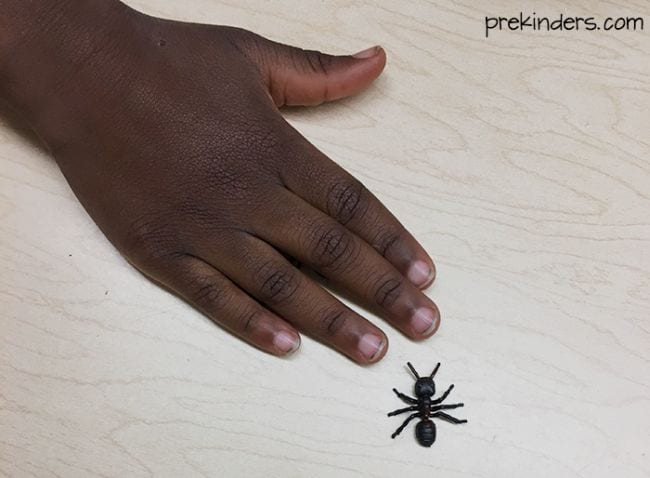Subtraction activities like this one are a fun way to introduce the idea to little learners. Start by laying a few small objects on a table. Next, cover some with your hand. Then, ask how many are left and how many you’re hiding. Simple, but effective.

## 20. Stack cups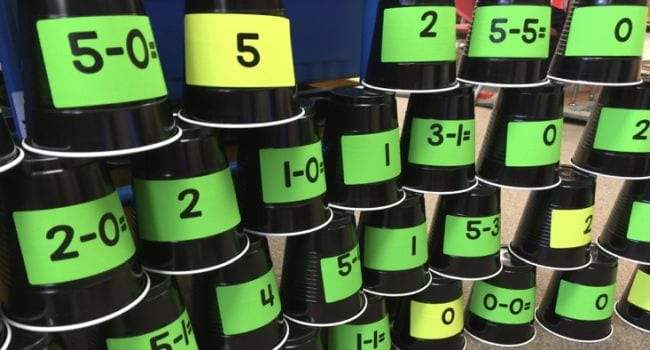Kids get such a kick out of stacking cups. Work subtraction practice into the mix by labeling the cups with equations and solutions, then challenge students to stack the cups to show the right answers.

## 21. Use pool noodles and gophersYour students will love this fun activity that builds math skills with puppets. Pop these darling gophers on a stick into the pool noodle, then take a few away to solve the problem.

## 22. Solve and play bingoFirst, kids work out the correct answers to the two-digit subtraction problems on this free printable. Then, they cut them out and paste them onto their bingo board. Finally, play a game of bingo together!

## 23. Play gumball unscramble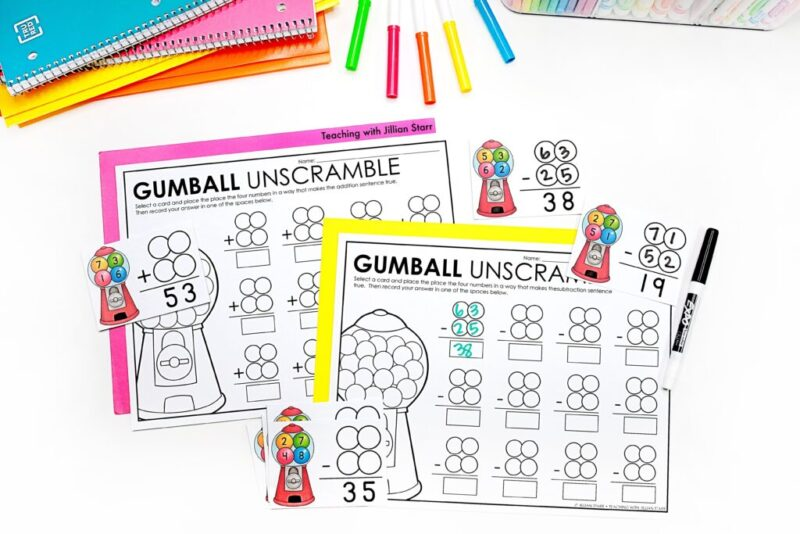Pull a subtraction card, then use the four digits in the gumball machine to create a number sentence that equals the solution at the bottom of the equation.

## 24. Toss some beanbags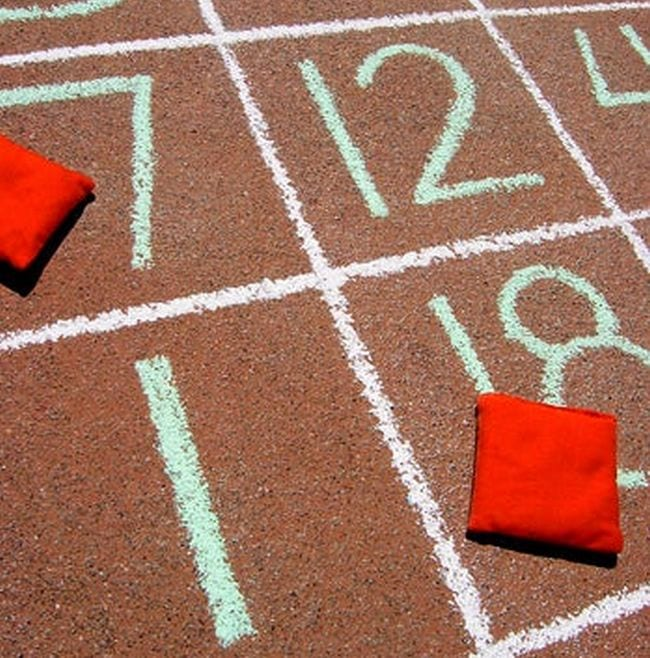Write a grid of randomized numbers on the sidewalk (or tape one off on your classroom floor). A student tosses two bean bags onto numbers, then makes a subtraction equation out of their results.

## 25. Use a zipper slider bag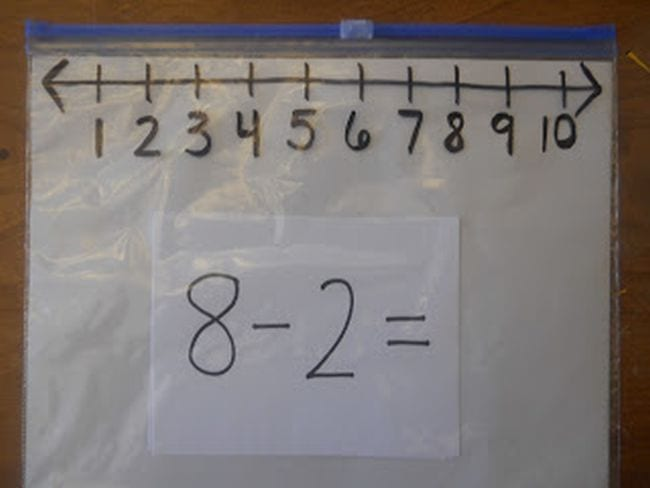A zipper bag number line? Genius! This clever idea is a useful tool for lots of subtraction activities. (Check out more number line ideas here.)

## 26. Slide beads on pipe cleaners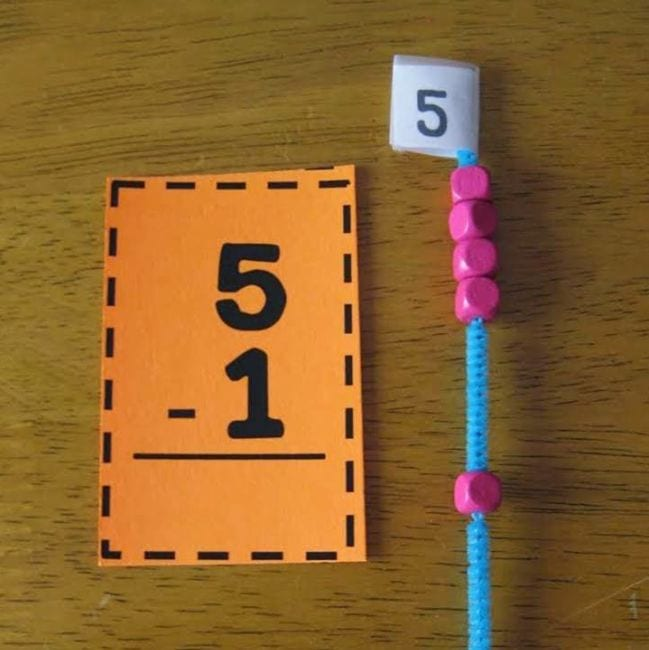Make some handy math manipulatives with pipe cleaners and beads. Slide the beads to see subtraction equations in action!

## 27. Build a wrecking ball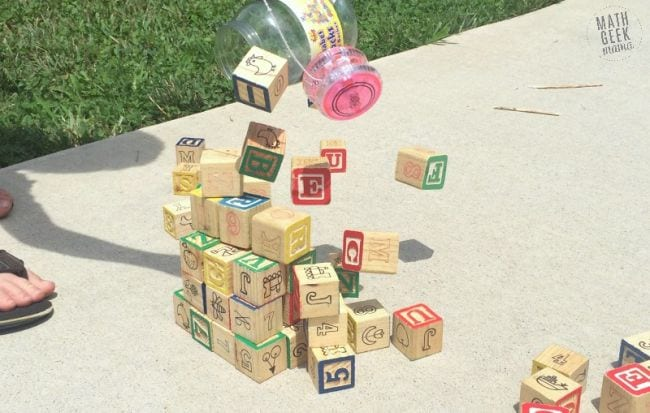One more chance to create a little mayhem! Similar to the bowling activity above, kids stack a number of blocks, then knock them down and see how many remain. Noisy, but fun!

## 28. Learn the subtraction rhymes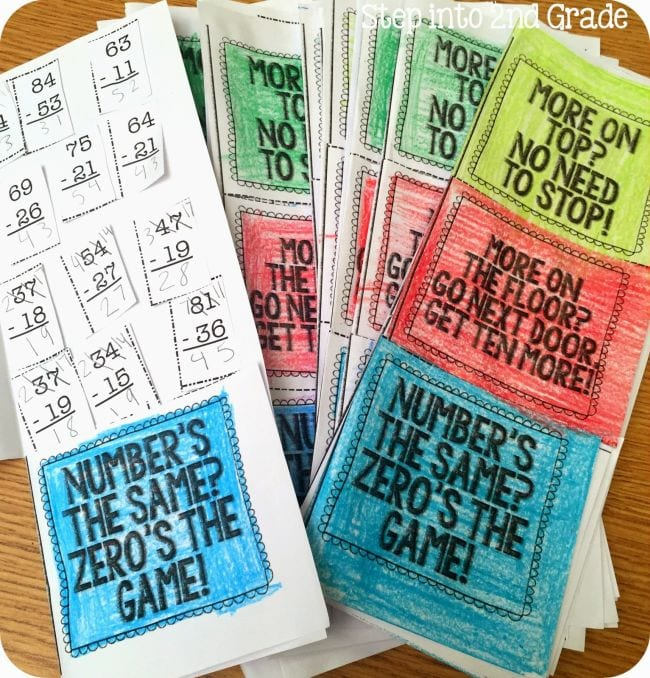These rhymes will aid kids working on more advanced subtraction activities, helping them remember when they need to “borrow” from the next place value column—and when they don’t.

### Plus, 75+ Awesome Websites for Teaching and Learning Math.## You Might Also Like